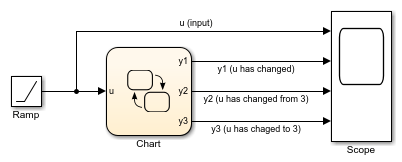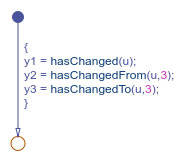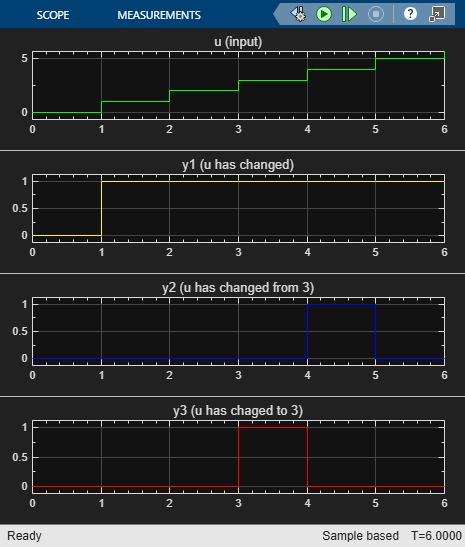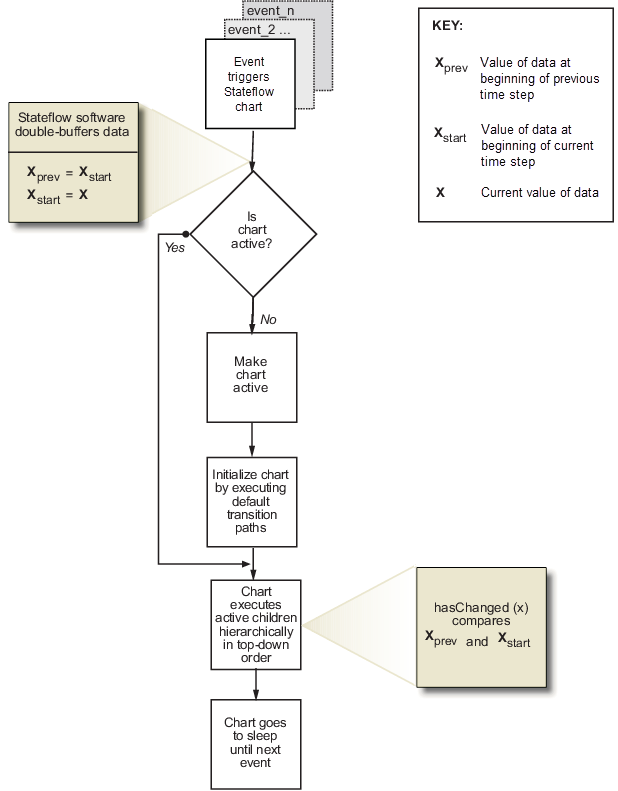Main Content

## Detect Changes in Data Values

Stateflow® charts can detect changes in the values of data and expressions between time steps. You can use change detection operators to determine when a variable changes to or from a value.

To generate an implicit local event when the chart sets the value of a variable, use the `change` operator. For more information, see Control Chart Behavior by Using Implicit Events.

### Change Detection Operators

To detect changes in Stateflow data, use the operators listed in this table.

OperatorSyntaxDescriptionExample
`hasChanged``tf = hasChanged(data_name)`Returns 1 (`true`) if the value of `data_name` at the beginning of the current time step is different from the value of `data_name` at the beginning of the previous time step. Otherwise, the operator returns 0 (`false`).

Transition out of state if any element of the matrix `M` has changed value since the last time step or input event.

`[hasChanged(M)]`

Transition out of state if the element in row 1 and column 3 of the matrix `M` has changed value since the last time step or input event.

In charts that use MATLAB® as the action language, use:

`[hasChanged(M(1,3))]`

In charts that use C as the action language, use:

`[hasChanged(M)]`
`hasChangedFrom``tf = hasChangedFrom(data_name,value)`Returns 1 (`true`) if the value of `data_name` was equal to the specified `value` at the beginning of the previous time step and is a different value at the beginning of the current time step. Otherwise, the operator returns 0 (`false`).

Transition out of state if the previous value of the structure `struct` was equal to `structValue` and any field of `struct` has changed value since the last time step or input event.

`[hasChangedFrom(struct,structValue)]`
`hasChangedTo``tf = hasChangedTo(data_name,value)`Returns 1 (`true`) if the value of `data_name` was not equal to the specified `value` at the beginning of the previous time step and is equal to `value` at the beginning of the current time step. Otherwise, the operator returns 0 (`false`).

Transition out of state if the structure field `struct.field` has changed to the value `5` since the last time step or input event.

`[hasChangedTo(struct.field,5)]`

Note

If multiple input events occur in the same time step, these operators can detect changes in data value between input events.

#### Example of Chart with Change Detection

This model shows how the operators `hasChanged`, `hasChangedFrom`, and `hasChangedTo` detect specific changes in an input signal. In this example, a Ramp (Simulink) block sends a discrete, increasing time signal to a chart.The model uses a fixed-step solver with a step size of 1. The signal increments by 1 every time step. The chart analyzes the input signal `u` for these changes:

• Any change from the previous time step

• A change to the value 3

• A change from the value 3

To check the signal, the chart calls three change detection operators in a transition action. The chart outputs the return values as `y1`, `y2`, and `y3`.During simulation, the Scope (Simulink) block shows the input and output signals for the chart.

• The value of `u` increases by 1 every time step.

• The value of `y1` changes from 0 to 1 at time t = 1. The value of `y1` remains 1 because `u` continues to change at each subsequent time step.

• The value of `y2` changes from 0 to 1 at time t = 4 when the value of `u` changes from 3 to 4. The value of `y2` returns to 0 after one time step.

• The value of `y3` changes from 0 to 1 at time t = 3 when the value of `u` changes from 2 to 3. The value of `y3` returns to 0 after one time step.#### Limitations of Change Detection

The type of Stateflow chart determines the scope of the data supported for change detection:

• Standalone Stateflow charts in MATLAB: `Local` only

• In Simulink® models, charts that use MATLAB as the action language: `Input` only

• In Simulink models, charts that use C as the action language: `Input`, `Output`, `Local`, or ```Data Store Memory```

The argument `data_name` can be:

• A scalar variable.

• A matrix or an element of a matrix.

• If `data_name` is a matrix, the operator returns `true` when it detects a change in any element of `data_name`.

• Index elements of a matrix by using numbers or expressions that evaluate to a constant integer. See Supported Operations for Vectors and Matrices.

• A structure or a field in a structure.

• If `data_name` is a structure, the change detection operator returns `true` when it detects a change in any field of `data_name`.

• Index fields in a structure by using dot notation. See Index and Assign Values to Stateflow Structures.

• Any valid combination of structure fields or matrix elements.

The argument `data_name` cannot be a nontrivial expression or a custom code variable.

Note

Standalone charts in MATLAB do not support change detection on an element of a matrix or a field in a structure.

For the `hasChangedFrom` and `hasChangedTo` operators, the argument `value` can be any expression that resolves to a value that is comparable with `data_name`.

• If `data_name` is a scalar, then `value` must resolve to a scalar value.

• If `data_name` is a matrix, then `value` must resolve to a matrix value with the same dimensions as `data_name`.

Alternatively, in a chart that uses C as the action language, `value` can resolve to a scalar value. The chart uses scalar expansion to compare `data_name` to a matrix whose elements are all equal to the value specified by `value`. See Assign Values to All Elements of a Matrix.

• If `data_name` is a structure, then `value` must resolve to a structure value whose field specification matches `data_name` exactly.

When you use change detection operators in charts while row-major array layout is enabled, code generation produces an error. Before generating code in charts that use change detection operators, enable column-major array layout. See Select Array Layout for Matrices in Generated Code.

### Implementation of Change Detection

A chart detects changes in chart data by evaluating values at time step boundaries. The chart compares the value at the beginning of the previous execution step with the value at the beginning of the current execution step.

For example, when you invoke the `hasChanged` operator with an argument of `x`, the Stateflow chart double-buffers the values of `x` in local variables.

Local BufferDescription
`x_prev`

Value of data `x` at the beginning of the last time step

`x_start`

Value of data `x` at the beginning of the current time step

To detect changes, the chart double-buffers data values after an event triggers the chart but before the chart begins execution. If the values of `xprev` and `xstart` match, the change detection operator returns `false` (to indicate that no change occurred); otherwise, it returns `true` (to indicate a change). This diagram places these tasks in the context of the chart life cycle.#### Transient Value Changes in Local Data

The change detection operators attempt to filter out transient changes in local chart variables by evaluating their values only at time boundaries. The chart evaluates the specified local variable only once at the end of the execution step. The return value of the change detection operators remains constant even if the value of the local variable fluctuates within a given time step. For example, suppose that in the current time step, the local variable `temp` changes from its value at the previous time step but then reverts to the original value. The operator `hasChanged(temp)` returns `false` for the next time step, indicating that no change occurred.

#### Detect Value Changes Between Input Events or Super Step Iterations

When multiple input events occur in the same time step, or when you enable super step semantics, the chart updates the `xprev` and `xstart` buffers every time it executes. The chart detects changes in value between input events and super step iterations, even if the changes occur more than once in a given time step. For more information, see Use Events to Execute Charts and Super Step Semantics.

## SupportGet trial now